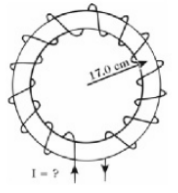# Problem: An ideal toroidal solenoid containing 1000 equally spaced coils is shown in the Figure below. How large must the current I be so that the magnetic field within the coils at a distance of 20.0 cm from the center is 0.0250 T?

###### Problem Details

An ideal toroidal solenoid containing 1000 equally spaced coils is shown in the Figure below. How large must the current I be so that the magnetic field within the coils at a distance of 20.0 cm from the center is 0.0250 T?